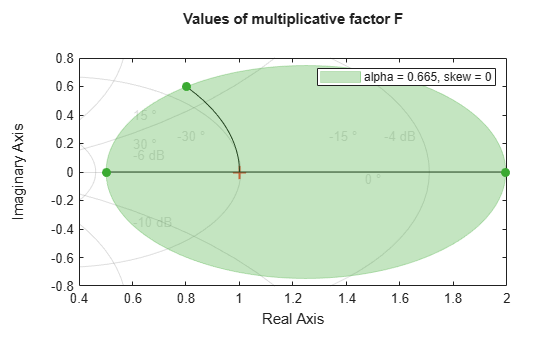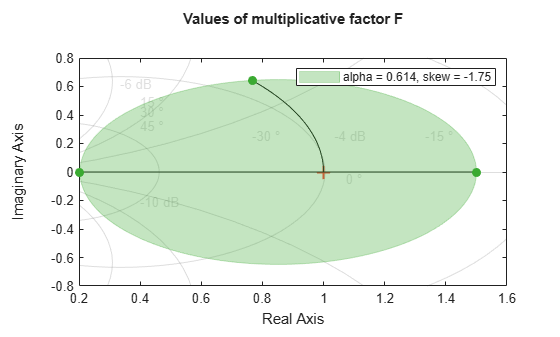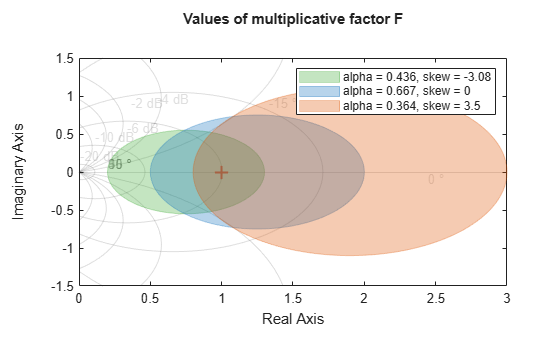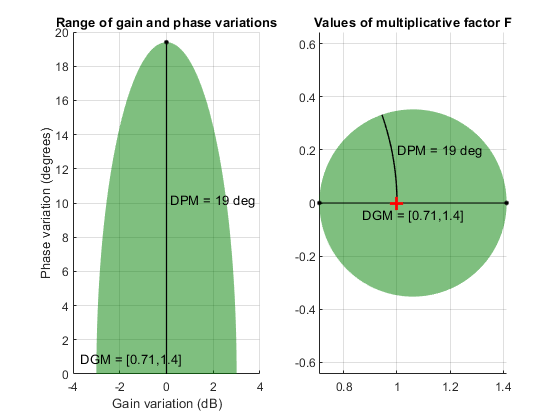# gm2dm

Convert disk-based gain margin to disk size and skew

Since R2020a

## Syntax

``[alpha,sigma] = gm2dm(DGM)``
``[alpha,sigma] = gm2dm(GM)``

## Description

`umargin` and `diskmargin` model gain and phase variation as a multiplicative factor F(s) taking values in a disk centered on the real axis. The disk is described by two parameters: ɑ, which sets the size of the variation, and σ, or skew, which biases the gain variation toward increase or decrease. (See Algorithms for more details about this model.) The disk can alternatively be described by its real-axis intercepts `DGM = [gmin,gmax]`, which represent the relative amount of gain variation around the nominal value F = 1. Use `gm2dm` and `dm2gm` to convert between the ɑ,σ values and the disk-based gain margin `DGM = [gmin,gmax]` that describe the same disk.

example

````[alpha,sigma] = gm2dm(DGM)` returns the disk size `alpha` and skew `sigma` corresponding to the disk-based gain margin `DGM`. The gain margin `DGM` is a vector of the form `[gmin,gmax]`. ```

example

````[alpha,sigma] = gm2dm(GM)` is the same as `gm2dm([1/GM,GM])`. This syntax returns the disk size for gain that can increase or decrease by a factor `GM`. This syntax always returns `sigma` = 0.```

## Examples

collapse all

Compute the disk size α of the disk that represents a gain variation of ±6 dB, that is, gain that can increase or decrease by about a factor of 2.

```GM = db2mag(6); [alpha,sigma] = gm2dm(GM)```
```alpha = 0.6646 ```
```sigma = 0 ```

For symmetric gain variations, the skew `sigma` is 0. Examine the disk corresponding to this gain variation.

`diskmarginplot(alpha,sigma,'disk')`The disk that captures gain variations of a factor of two in either direction also models phase variations of ±37°.

Determine the disk size and skew needed to capture gain variations between 80% and 150% of nominal and phase variation between –20 and +40 degrees. First, use `getDGM` to find `DGM = [gmin,gmax]` that describes a disk that captures these target ranges.

`DGM = getDGM([0.8,1.5],[-20,40],'tight')`
```DGM = 1×2 0.2031 1.5000 ```

Now use `gm2dm` to convert that disk-based gain variation into the α,σ parameterization of the disk.

`[alpha,sigma] = gm2dm(DGM)`
```alpha = 0.6145 ```
```sigma = -1.7451 ```

For the modeled gain and phase variations, the skew is less than zero because the disk-based gain range `DGM = [0.2 1.5]` includes more gain decrease than increase.

`diskmarginplot(alpha,sigma,'disk')`Determine the disk size and skew of the disks which capture gain ranges [0.2,1.3], [0.5,2] and [0.8,3].

```GainRange1 = [0.2,1.3]; GainRange2 = [0.5,2]; GainRange3 = [0.8,3];```

For the gain ranges above, compute the disk-based gain margin.

`[alpha,sigma] = gm2dm([GainRange1;GainRange2;GainRange3])`
```alpha = 3×1 0.4364 0.6667 0.3636 ```
```sigma = 3×1 -3.0833 0 3.5000 ```

For the vector `sigma`, the first entry is negative because first entry of `DGM` has bias toward gain decrease. Similarly, second entry is zero because of balanced gain variation and third entry is positive because of bias toward gain increase. The plot shows the disks corresponding to range of gain variations specified above.

`diskmarginplot(alpha,sigma,'disk')`## Input Arguments

collapse all

Range of relative gain variation, specified as a two-element vector of the form `[gmin,gmax]`, where `gmin` < 1 and `gmax` > 1. For instance, `DGM = [0.8 1.5]` represents a gain that can vary between 80% and 150% of its nominal value (that is, change by a factor between 0.8 and 1.5). `gmin` can be negative, defining a range of relative gain variation that includes a change in sign.

The range `[gmin,gmax]` describes a disk of gain and phase uncertainty where the gain can vary by `[gmin,gmax]` and the phase can vary by an amount determined by the disk geometry. The `gm2dm` command finds the disk size `alpha` and skew `sigma` that parameterize this disk. For more information about the disk-based uncertainty model, see Algorithms.

You can obtain `DGM` from desired gain and phase variations (or margins) using `getDGM`. The `GainMargin` field of the output structures of the `diskmargin` command is also a disk-based gain range of this form. .

To get `alpha` and `sigma` corresponding to multiple gain variation ranges at once, specify `DGM` as a two-column matrix of the form `[gmin1,gmax1; ...;gminN,gmaxN]`, where each row is a corresponding disk-based gain range.

Amount of gain increase or decrease in absolute units, specified as a real scalar or a vector.

• If `GM` is a real scalar, then `gm2dm` returns the disk size `alpha` corresponding to the symmetric gain variation in the range `[1/GM,GM]`. For instance, `GM` = 2 specifies a gain that can increase or decrease by a factor of 2. For such symmetric gain variation, the skew `sigma` is zero.

• If `GM` is a vector of form `[GM1;...;GMN]`, the function returns `alpha` as a column vector of the corresponding disk sizes.

## Output Arguments

collapse all

Disk size of the uncertainty corresponding to the input gain range, returned as a scalar or vector. Disk-based gain-margin analysis represents gain and phase variation as a multiplicative uncertainty F, which is a disk of values containing F = 1, corresponding to the nominal value of the system. The disk is parameterized by `alpha`, which sets the size of the disk, and `sigma`, which biases the gain variation toward gain increase or decrease. See Algorithms for details about the meaning of `alpha`.

If `DGM` is a two-column matrix or `GM` is a column vector, then `alpha` is a vector of the form `[alpha1;...;alphaN]` of the corresponding disk sizes.

Skew of the modeled uncertainty disk, returned as a scalar or vector. The skew biases the modeled gain variation toward gain increase or decrease.

• `sigma` = 0 for a balanced gain range `[gmin,gmax]`, with `gmin = 1/gmax`.

• `sigma` is positive for a varying gain that can increase more than it can decrease, `gmax > 1/gmin`.

• `sigma` is negative for a varying gain that can decrease more than it can increase, `gmin < 1/gmax`.

The more the gain range is biased, the larger the absolute value of `sigma`. For a scalar gain variation input `GM`, the skew `sigma` is always zero. For additional details about the meaning of `sigma`, see Algorithms.

If `DGM` is a two-column matrix, then `sigma` is a vector of the form `[sigma1;...;sigmaN]` of the corresponding skew values.

## Algorithms

`umargin` and `diskmargin` model gain and phase variations in an individual feedback channel as a frequency-dependent multiplicative factor F(s) multiplying the nominal open-loop response L(s), such that the perturbed response is L(s)F(s). The factor F(s) is parameterized by:

`$F\left(s\right)=\frac{1+\alpha \left[\left(1-\sigma \right)/2\right]\delta \left(s\right)}{1-\alpha \left[\left(1+\sigma \right)/2\right]\delta \left(s\right)}.$`

In this model,

• δ(s) is a gain-bounded dynamic uncertainty, normalized so that it always varies within the unit disk (||δ|| < 1).

• ɑ sets the amount of gain and phase variation modeled by F. For fixed σ, the parameter ɑ controls the size of the disk. For ɑ = 0, the multiplicative factor is 1, corresponding to the nominal L.

• σ, called the skew, biases the modeled uncertainty toward gain increase or gain decrease.

The factor F takes values in a disk centered on the real axis and containing the nominal value F = 1. The disk is characterized by its intercept `DGM = [gmin,gmax]` with the real axis. `gmin` < 1 and `gmin` > 1 are the minimum and maximum relative changes in gain modeled by F, at nominal phase. The phase uncertainty modeled by F is the range `DPM = [-pm,pm]` of phase values at the nominal gain (|F| = 1). For instance, in the following plot, the right side shows the disk F that intersects the real axis in the interval [0.71,1.4]. The left side shows that this disk models a gain variation of ±3 dB and a phase variation of ±19°.

```DGM = [0.71,1.4] F = umargin('F',DGM) plot(F)````gm2dm` and `gm2dm` converts between these two ways of specifying a disk of multiplicative gain and phase uncertainty: a gain-variation range of the form `DGM = [gmin,gmax]`, and the ɑ,σ parameterization of the corresponding disk.

For further details about the uncertainty model for gain and phase variations, see Stability Analysis Using Disk Margins.

## Version History

Introduced in R2020a